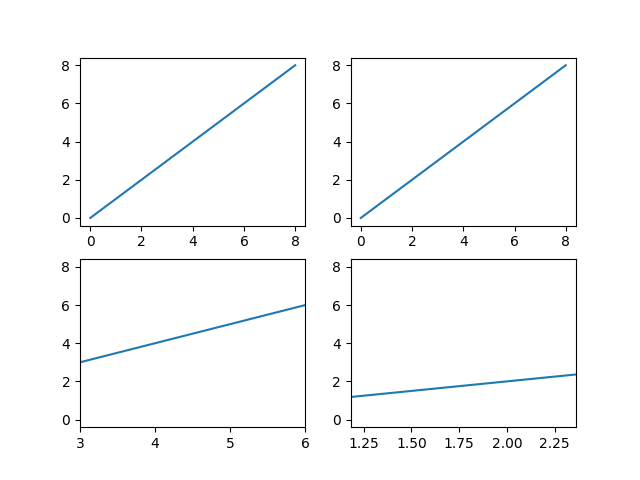# Inches and Centimeters¶

The example illustrates the ability to override default x and y units (ax1) to inches and centimeters using the `xunits` and `yunits` parameters for the `plot` function. Note that conversions are applied to get numbers to correct units.

This example requires `basic_units.py````from basic_units import cm, inch
import matplotlib.pyplot as plt
import numpy as np

cms = cm * np.arange(0, 10, 2)

fig, axs = plt.subplots(2, 2)

axs[0, 0].plot(cms, cms)

axs[0, 1].plot(cms, cms, xunits=cm, yunits=inch)

axs[1, 0].plot(cms, cms, xunits=inch, yunits=cm)
axs[1, 0].set_xlim(3, 6)  # scalars are interpreted in current units

axs[1, 1].plot(cms, cms, xunits=inch, yunits=inch)
axs[1, 1].set_xlim(3*cm, 6*cm)  # cm are converted to inches

plt.show()
```

Keywords: matplotlib code example, codex, python plot, pyplot Gallery generated by Sphinx-Gallery